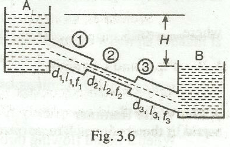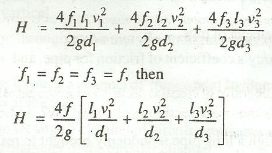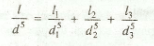# Pipes in Series or Compound Pipes

How do pipes work in series as taught to mechanical engineers in fluid mechanics? What is the difference between pipes in parallel and pipes in series?

Figure below shows a system of pipes in series:The difference of water level in the two tanks A and B is given byIf a compound pipe is to be replaced by a pipe of uniform diameter (known as equivalent pipe),then the loss of head and discharge of both the pipes should be same. The uniform diameter (d) of the equivalent pipe may be obtained from the following relation:where
l = Length of the equivalent pipe = l1 + l2 + l3

Read more about pipes in parallel to learn the difference between both these techniques.